# Angle Between Two Intersecting Lines and Shortest Distance JEE Notes | EduRev

## JEE : Angle Between Two Intersecting Lines and Shortest Distance JEE Notes | EduRev

The document Angle Between Two Intersecting Lines and Shortest Distance JEE Notes | EduRev is a part of the JEE Course Mathematics (Maths) Class 12.
All you need of JEE at this link: JEE

Angle Between Two Intersecting Lines

If l(x1, m1, n1) and l(x2, m2, n2) be the direction cosines of two given lines, then the angle θ between them is given by

cos θ = l112 + m1m2 + n1n2

(i) The angle between any two diagonals of a cube is cos-1 (1 / 3).

(ii) The angle between a diagonal of a cube and the diagonal of a face (of the cube is cos-1(√2 / 3)

Straight Line in Space

The two equations of the line ax + by + cz + d = 0 and a’ x + b’ y + c’ z + d’ = 0 together represents a straight line.

1. Equation of a straight line passing through a fixed point A(x1, y1, z1) and having direction ratios a, b, c is given by

x – x1 / a = y – y1 / b = z – z1 / c, it is also called the symmetrically form of a line.

Any point P on this line may be taken as (x1 + λa, y1 + λb, z1 + λc), where λ ∈ R is parameter. If a, b, c are replaced by direction cosines 1, m, n, then λ, represents distance of the point P from the fixed point A.

2. Equation of a straight line joining two fixed points A(x1, y1, z1) and B(x2, y2, z2) is given by

x – x1 / x2 – x1 = y – y1 / y2 – y1 = z – z1 / z2 – z1

3. Vector equation of a line passing through a point with position vector a and parallel to vector b is r = a + λ b, where A, is a parameter.

4. Vector equation of a line passing through two given points having position vectors a and b is r = a + λ (b – a) , where λ is a parameter.

5. (a) The length of the perpendicular from a point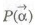on the line r – a + λ b is given by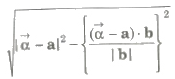(b) The length of the perpendicular from a point P(x1, y1, z1) on the line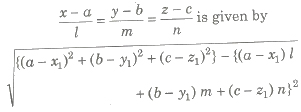where, 1, m, n are direction cosines of the line.

6. Skew Lines Two straight lines in space are said to be skew lines, if they are neither parallel nor intersecting.

7. Shortest Distance If l1 and l2 are two skew lines, then a line perpendicular to each of lines 4 and 12 is known as the line of shortest distance.

If the line of shortest distance intersects the lines l1 and l2 at P and Q respectively, then the distance PQ between points P and Q is known as the shortest distance between l1 and l2.

8. The shortest distance between the lines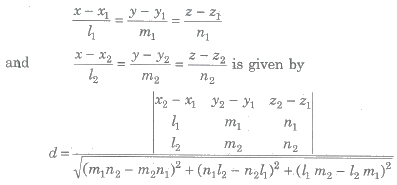9. The shortest distance between lines r = a1 + λb1 and r = a2 + μb2 is given by10. The shortest distance parallel lines r = a1 + λb1 and r = a2 + μb2 is given by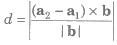11. Lines r = a1 + λb1 and r = a2 + μb2 are intersecting lines, if (b1 * b2) * (a2 – a1) = 0.

12. The image or reflection (x, y, z) of a point (x1, y1, z1) in a plane ax + by + cz + d = 0 is given by

x – x1 / a = y – y1 / b = z – z1 / c = – 2 (ax1 + by1 + cz1 + d) / a2 + b2 + c2

13. The foot (x, y, z) of a point (x1, y1, z1) in a plane ax + by + cz + d = 0 is given by

x – x1 / a = y – y1 / b = z – z1 / c = – (ax1 + by1 + cz1 + d) / a2 + b2 + c2

14. Since, x, y and z-axes pass through the origin and have direction cosines (1, 0, 0), (0, 1, 0) and (0, 0, 1), respectively. Therefore, their equations are

x – axis : x – 0 / 1 = y – 0 / 0 = z – 0 / 0

y – axis : x – 0 / 0 = y – 0 / 1 = z – 0 / 0

z – axis : x – 0 / 0 = y – 0 / 0 = z – 0 / 1

Offer running on EduRev: Apply code STAYHOME200 to get INR 200 off on our premium plan EduRev Infinity!

## Mathematics (Maths) Class 12

209 videos|222 docs|124 tests

,

,

,

,

,

,

,

,

,

,

,

,

,

,

,

,

,

,

,

,

,

;